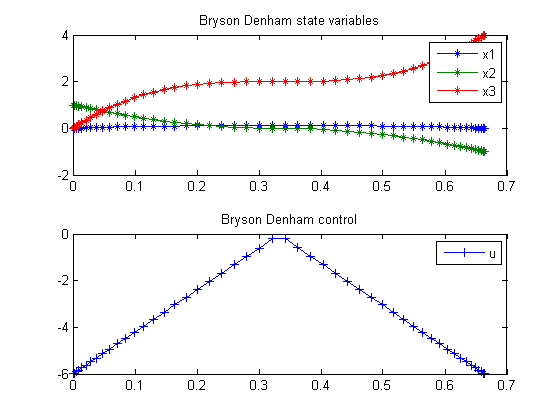17  Bryson-Denham Problem

17.1  Problem description

Standard formulation for Bryson-Denham

Reference: 

17.2  Problem setup

toms t t_f
p = tomPhase('p', t, 0, t_f, 50);
setPhase(p);

x10 = 0; x20 = 1;
x30 = 0; x1f = 0; x2f = -1;
x1min = -10;   x1max = 10;    x2min = x1min;
x2max = x1max; x3min = x1min; x3max = x1max;

tomStates x1 x2 x3
tomControls u

% Initial guess
x0 = {t_f == 0.5
icollocate({
x1 == x10+(x1f-x10)*t/t_f
x2 == x20+(x2f-x20)*t/t_f
x3 == x30
})
collocate(u==0)};

% Box constraints
cbox = {0.001 <= t_f <= 50
0      <= mcollocate(x1) <= 1/9
x2min  <= mcollocate(x2) <= x2max
x3min  <= mcollocate(x3) <= x3max
-5000  <= collocate(u)   <= 5000};

% Boundary constraints
cbnd = {initial({x1 == x10; x2 == x20; x3 == x30})
final({x1 == x1f; x2 == x2f})};

% ODEs and path constraints
ceq = collocate({
dot(x1) == x2
dot(x2) == u
dot(x3) == u.^2/2});

% Objective
objective = final(x3);

17.3  Solve the problem

options = struct;
options.name = 'Bryson Denham';
solution = ezsolve(objective, {cbox, cbnd, ceq}, x0, options);
t  = subs(collocate(t),solution);
x1 = subs(collocate(x1),solution);
x2 = subs(collocate(x2),solution);
x3 = subs(collocate(x3),solution);
u  = subs(collocate(u),solution);
Problem type appears to be: lpcon
Starting numeric solver
===== * * * =================================================================== * * *
TOMLAB - Tomlab Optimization Inc. Development license  999001. Valid to 2011-02-05
=====================================================================================
Problem: ---  1: Bryson Denham                  f_k       4.000021208621198800
sum(|constr|)      0.000000174861447145
f(x_k) + sum(|constr|)      4.000021383482645900
f(x_0)      0.000000000000000000

Solver: snopt.  EXIT=0.  INFORM=1.
SNOPT 7.2-5 NLP code
Optimality conditions satisfied

FuncEv    1 ConstrEv   63 ConJacEv   63 Iter   60 MinorIter  260
CPU time: 0.906250 sec. Elapsed time: 0.922000 sec.

17.4  Plot result

subplot(2,1,1)
plot(t,x1,'*-',t,x2,'*-',t,x3,'*-');
legend('x1','x2','x3');
title('Bryson Denham state variables');

subplot(2,1,2)
plot(t,u,'+-');
legend('u');
title('Bryson Denham control');